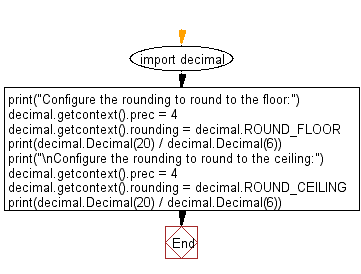﻿ Python: Configure the rounding to round to the floor, ceiling - w3resource# Python: Configure the rounding to round to the floor, ceiling

## Python module: Exercise-4 with Solution

Write a Python program to configure the rounding to round to the floor, ceiling. Use decimal.ROUND_FLOOR, decimal.ROUND_CEILING

Sample Solution:

Python Code:

``````import decimal
print("Configure the rounding to round to the floor:")
decimal.getcontext().prec = 4
decimal.getcontext().rounding = decimal.ROUND_FLOOR
print(decimal.Decimal(20) / decimal.Decimal(6))
print("\nConfigure the rounding to round to the ceiling:")
decimal.getcontext().prec = 4
decimal.getcontext().rounding = decimal.ROUND_CEILING
print(decimal.Decimal(20) / decimal.Decimal(6))
```
```

Sample Output:

```Configure the rounding to round to the floor:
3.333

Configure the rounding to round to the ceiling:
3.334
```

Flowchart:## Visualize Python code execution:

The following tool visualize what the computer is doing step-by-step as it executes the said program:

Python Code Editor:

Have another way to solve this solution? Contribute your code (and comments) through Disqus.

What is the difficulty level of this exercise?

Test your Python skills with w3resource's quiz

﻿

## Python: Tips of the Day

For-else construct useful when searched for something and find it:

```# For example assume that I need to search through a list and process each item until a flag item is found and
# then stop processing. If the flag item is missing then an exception needs to be raised.

for i in mylist:
if i == theflag:
break
process(i)
else:
raise ValueError("List argument missing terminal flag.")
```